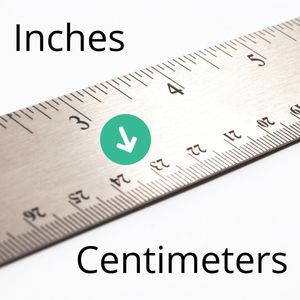## Inches to Centimeters Calculator

Enter Inches in the top textbox. Click Convert. Results appear below in Centimeters (rounded down).

Inches (in)
Centimeters (cm)## Inches to Centimeters List

10 inches to centimeters equals 25.
20 inches to centimeters equals 50.
30 inches to centimeters equals 76.
40 inches to centimeters equals 101.
50 inches to centimeters equals 127.
60 inches to centimeters equals 152.
70 inches to centimeters equals 177.
80 inches to centimeters equals 203.
90 inches to centimeters equals 228.
100 inches to centimeters equals 254.